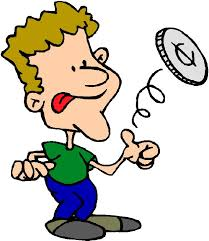# TXT meYou flip a fair coin several times.

Find the expected value for the number of flips you'll need to make in order to see the pattern TXT, where T is tails, and X is either heads or tails.

If the expectation value can be expressed as $\frac ab$, where $a$ and $b$ are coprime positive integers, find $a+b$.


Clarification: You are looking for the pattern TTT or THT. That is, you will keep flipping until you see one of these two patterns, and then count the number of flips you made.

Other Expected Value Quizzes

×